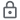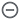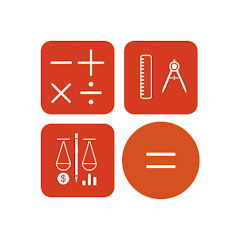# Calc Plus - Simple Calculator

50+Everyone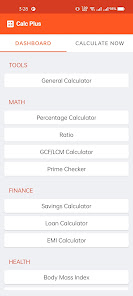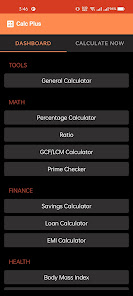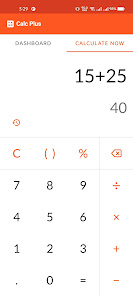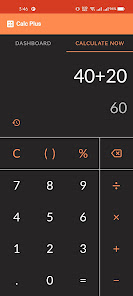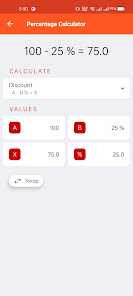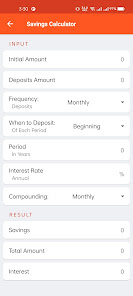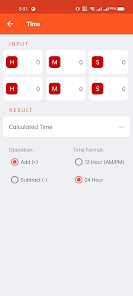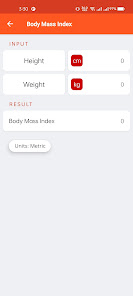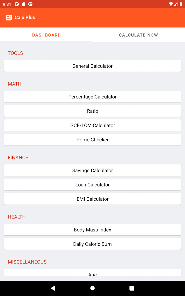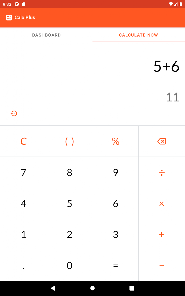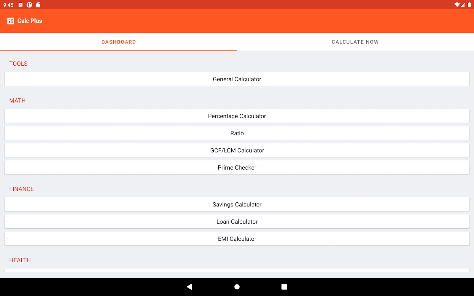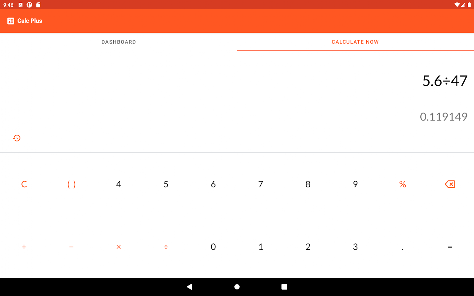Calc Plus, a calculator pack application for calculating at ease, along with other types of calculators like mathematics, finance, health, unit converters and so on. Enabling features like keeping calculations history lets you take a break at any time during calculations. You can just come back later and restart from where you left. A simple calculator app with a clean interface and practical functions!
By using this calculator app, you can solve your daily life problems. Each calculator in this app is too easy to apply. Most of the common problems’ quick calculation you need are at your fingertips when you use this app.
General Calculator
Quick calculations for math with addition, subtraction, division and multiplication. Always continue where you left. Your previous calculations will be autosaved.
Percentage Calculator
Calculate discount, simple percentage, increase/decrease in percentage and percentage of one value of another. Just switch between the calculation modes as per needs.
Ratio Calculator
Easily calculate the ratio of any numerical value. It also has 2 other modes to calculate numerator and denominator.
GCF/LCM Calculator
Calculating “Greatest Common Factor” and “Least Common Multiple” is now one step away, simply add your numbers and get the results instantly.
Prime Calculator
Can’t find out if a number is prime or not? Use our prime calculator to determine whether a number is prime. It also generates the next prime number which can be regenerated by one click.
Savings Calculator
Want to plan savings for the future? Try our savings calculator to calculate your future savings at ease.
Loan Calculator
Calculate your loan before taking it. You can calculate your loan payment by monthly, quarterly, yearly and so on.
EMI Calculator
Our Equated Monthly Instalment calculator can help you to calculate your monthly EMI before buying any product. You can calculate EMI monthly, quarterly and so on.
Body Mass Index
For maintaining health, our BMI calculator provides not just a BMI ratio but also tells your current health condition.
Calorie Calculator
Thinking of losing or gaining some weight? Our calorie calculator gives you the calorie to burn on the basis of your daily activity level.
Age Calculator
Countdown next birthday, age, newborn baby’s age with our Age Calculator.
Date Calculator
Track any date in the past or future by date calculator. You can also calculate days before or after a particular date.
Time Calculator
Simply add or subtract two times using our time calculator both in 12 hour and 24 hour format.
Unit Converters
Now convert your daily necessary units from calc plus that includes unit types of length, area, digital storage, energy, fuel consumption, mass, power, pressure, temperature, time, torque, volume. Also keep keep track your recent conversions and control what you want to keep.
Updated on
Nov 12, 2022

## Data safety

Safety starts with understanding how developers collect and share your data. Data privacy and security practices may vary based on your use, region, and age. The developer provided this information and may update it over time.This app may share these data types with third parties
App info and performance and Device or other IDsNo data collected